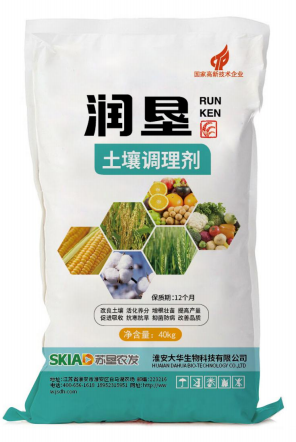• 水产专用
• 大量元素水溶肥料
• 含腐殖酸水溶肥料
• 含氨基酸水溶肥料
• 发酵菌剂
• 沼气发酵菌剂
• 肥水专用
• 净水剂
• 发酵增产剂
• 生物反应堆菌剂
• 秸秆饲料发酵剂
• 酵素菌速腐剂
• 废菌料发酵剂
• 育苗基质
• 水稻育苗基质
• 蓝莓栽培基质
• 蔬菜育苗基质
• 生物有机肥
• 有机肥料
• 生物有机肥
• 食用菌专用
• 发酵增产剂
• 菇宝乐
• 联系我们
• 产品分类
• 发酵菌剂
• 生物反应堆菌剂
• 沼气发酵菌剂
• 肥水专用
• 发酵增产剂
• 秸秆饲料发酵剂
• 废菌料发酵剂
• 酵素菌速腐剂
• 净水剂
• 水溶肥料
• 大量元素水溶肥料
• 含腐殖酸水溶肥料
• 含氨基酸水溶肥料
• 育苗基质
• 蓝莓栽培基质
• 蔬菜育苗基质
• 水稻育苗基质
• 生物有机肥
• 有机肥料
• 生物有机肥
• 水产制剂
• 水产专用
• 食用菌专用
• 菇宝乐
• 发酵增产剂
• 园艺资材
• 有机肥料
• 水稻育苗基质
• 花盆
• 含氨基酸水溶肥料
• 菇宝乐
• 发酵增产剂
• 土壤修复剂
• 土壤调理剂
• 产品分类
• 发酵菌剂
• 水溶肥料
• 育苗基质
• 生物有机肥
• 食用菌专用
• 园艺资材
• 土壤调理剂
• 土壤修复剂
• 水产制剂
• 产品分类
• 土壤调理剂
• 土壤修复剂
• 水产制剂
• 水产专用
• 育苗基质
• 蓝莓栽培基质
• 蔬菜育苗基质
• 水稻育苗基质
• 发酵菌剂
• 发酵增产剂
• 肥水专用
• 沼气发酵菌剂
• 净水剂
• 生物反应堆菌剂
• 秸秆饲料发酵剂
• 废菌料发酵剂
• 酵素菌速腐剂
• 水溶肥料
• 大量元素水溶肥料
• 含氨基酸水溶肥料
• 含腐殖酸水溶肥料
• 生物有机肥
• 有机肥料
• 生物有机肥
• 食用菌专用
• 菇宝乐
• 发酵增产剂
• 园艺资材
• 菇宝乐
• 发酵增产剂
• 花盆
• 水稻育苗基质
• 含氨基酸水溶肥料
• 有机肥料
• 产品分类
• 发酵菌剂
• 发酵增产剂
• 沼气发酵菌剂
• 净水剂
• 生物反应堆菌剂
• 秸秆饲料发酵剂
• 废菌料发酵剂
• 酵素菌速腐剂
• 肥水专用
• 水溶肥料
• 大量元素水溶肥料
• 含腐殖酸水溶肥料
• 含氨基酸水溶肥料
• 育苗基质
• 蓝莓栽培基质
• 蔬菜育苗基质
• 水稻育苗基质
• 生物有机肥
• 有机肥料
• 生物有机肥
• 土壤调理剂
• 食用菌专用
• 菇宝乐
• 发酵增产剂
• 园艺资材
• 有机肥料
• 水稻育苗基质
• 花盆
• 含氨基酸水溶肥料
• 发酵增产剂
• 菇宝乐
• 土壤修复剂
• 水产制剂
• 水产专用
• 产品分类
• 土壤调理剂
• 土壤修复剂
• 水产制剂
• 水产专用
• 发酵菌剂
• 沼气发酵菌剂
• 肥水专用
• 净水剂
• 发酵增产剂
• 生物反应堆菌剂
• 秸秆饲料发酵剂
• 废菌料发酵剂
• 酵素菌速腐剂
• 水溶肥料
• 含氨基酸水溶肥料
• 含腐殖酸水溶肥料
• 大量元素水溶肥料
• 育苗基质
• 蓝莓栽培基质
• 蔬菜育苗基质
• 水稻育苗基质
• 生物有机肥
• 有机肥料
• 生物有机肥
• 食用菌专用
• 菇宝乐
• 发酵增产剂
• 园艺资材
• 含氨基酸水溶肥料
• 菇宝乐
• 发酵增产剂
• 水稻育苗基质
• 有机肥料
• 花盆
• 网站首页
• 关于我们
• 总经理致辞
• 企业文化
• 组织架构
• 公司概况
• 产品分类
• 土壤调理剂
• 水产制剂
• 水产专用
• 园艺资材
• 有机肥料
• 水稻育苗基质
• 花盆
• 含氨基酸水溶肥料
• 菇宝乐
• 发酵增产剂
• 育苗基质
• 蓝莓栽培基质
• 蔬菜育苗基质
• 水稻育苗基质
• 生物有机肥
• 有机肥料
• 生物有机肥
• 食用菌专用
• 发酵增产剂
• 菇宝乐
• 土壤修复剂
• 水溶肥料
• 大量元素水溶肥料
• 含腐殖酸水溶肥料
• 含氨基酸水溶肥料
• 发酵菌剂
• 废菌料发酵剂
• 酵素菌速腐剂
• 沼气发酵菌剂
• 肥水专用
• 净水剂
• 发酵增产剂
• 生物反应堆菌剂
• 秸秆饲料发酵剂
• 产品分类
• 发酵菌剂
• 沼气发酵菌剂
• 肥水专用
• 净水剂
• 发酵增产剂
• 生物反应堆菌剂
• 秸秆饲料发酵剂
• 废菌料发酵剂
• 酵素菌速腐剂
• 水溶肥料
• 大量元素水溶肥料
• 含腐殖酸水溶肥料
• 含氨基酸水溶肥料
• 育苗基质
• 蓝莓栽培基质
• 蔬菜育苗基质
• 水稻育苗基质
• 生物有机肥
• 有机肥料
• 生物有机肥
• 食用菌专用
• 菇宝乐
• 发酵增产剂
• 园艺资材
• 含氨基酸水溶肥料
• 有机肥料
• 花盆
• 菇宝乐
• 发酵增产剂
• 水稻育苗基质
• 土壤调理剂
• 土壤修复剂
• 水产制剂
• 水产专用
• 产品分类
• 园艺资材
• 有机肥料
• 水稻育苗基质
• 花盆
• 含氨基酸水溶肥料
• 菇宝乐
• 发酵增产剂
• 土壤调理剂
• 水产制剂
• 水产专用
• 土壤修复剂
• 发酵菌剂
• 生物反应堆菌剂
• 沼气发酵菌剂
• 肥水专用
• 发酵增产剂
• 秸秆饲料发酵剂
• 废菌料发酵剂
• 酵素菌速腐剂
• 净水剂
• 食用菌专用
• 菇宝乐
• 发酵增产剂
• 水溶肥料
• 大量元素水溶肥料
• 含腐殖酸水溶肥料
• 含氨基酸水溶肥料
• 育苗基质
• 蓝莓栽培基质
• 蔬菜育苗基质
• 水稻育苗基质
• 生物有机肥
• 生物有机肥
• 有机肥料
• 产品分类
• 食用菌专用
• 菇宝乐
• 发酵增产剂
• 水溶肥料
• 大量元素水溶肥料
• 含腐殖酸水溶肥料
• 含氨基酸水溶肥料
• 育苗基质
• 蓝莓栽培基质
• 蔬菜育苗基质
• 水稻育苗基质
• 生物有机肥
• 生物有机肥
• 有机肥料
• 园艺资材
• 有机肥料
• 水稻育苗基质
• 花盆
• 含氨基酸水溶肥料
• 菇宝乐
• 发酵增产剂
• 土壤调理剂
• 土壤修复剂
• 水产制剂
• 水产专用
• 发酵菌剂
• 废菌料发酵剂
• 酵素菌速腐剂
• 发酵增产剂
• 秸秆饲料发酵剂
• 生物反应堆菌剂
• 沼气发酵菌剂
• 肥水专用
• 净水剂
• 产品分类
• 发酵菌剂
• 沼气发酵菌剂
• 肥水专用
• 生物反应堆菌剂
• 净水剂
• 秸秆饲料发酵剂
• 废菌料发酵剂
• 酵素菌速腐剂
• 发酵增产剂
• 土壤修复剂
• 水溶肥料
• 大量元素水溶肥料
• 含腐殖酸水溶肥料
• 含氨基酸水溶肥料
• 育苗基质
• 蓝莓栽培基质
• 蔬菜育苗基质
• 水稻育苗基质
• 水产制剂
• 水产专用
• 食用菌专用
• 菇宝乐
• 发酵增产剂
• 园艺资材
• 有机肥料
• 水稻育苗基质
• 花盆
• 含氨基酸水溶肥料
• 菇宝乐
• 发酵增产剂
• 土壤调理剂
• 生物有机肥
• 生物有机肥
• 有机肥料
• 产品分类
• 育苗基质
• 蓝莓栽培基质
• 蔬菜育苗基质
• 水稻育苗基质
• 生物有机肥
• 有机肥料
• 生物有机肥
• 食用菌专用
• 菇宝乐
• 发酵增产剂
• 土壤调理剂
• 土壤修复剂
• 水产制剂
• 水产专用
• 园艺资材
• 水稻育苗基质
• 有机肥料
• 花盆
• 含氨基酸水溶肥料
• 菇宝乐
• 发酵增产剂
• 发酵菌剂
• 沼气发酵菌剂
• 肥水专用
• 净水剂
• 发酵增产剂
• 生物反应堆菌剂
• 秸秆饲料发酵剂
• 废菌料发酵剂
• 酵素菌速腐剂
• 水溶肥料
• 含腐殖酸水溶肥料
• 大量元素水溶肥料
• 含氨基酸水溶肥料
• 产品分类
• 土壤调理剂
• 土壤修复剂
• 水产制剂
• 水产专用
• 发酵菌剂
• 沼气发酵菌剂
• 肥水专用
• 净水剂
• 发酵增产剂
• 生物反应堆菌剂
• 秸秆饲料发酵剂
• 废菌料发酵剂
• 酵素菌速腐剂
• 水溶肥料
• 含氨基酸水溶肥料
• 含腐殖酸水溶肥料
• 大量元素水溶肥料
• 育苗基质
• 蓝莓栽培基质
• 蔬菜育苗基质
• 水稻育苗基质
• 生物有机肥
• 有机肥料
• 生物有机肥
• 食用菌专用
• 菇宝乐
• 发酵增产剂
• 园艺资材
• 花盆
• 菇宝乐
• 发酵增产剂
• 水稻育苗基质
• 含氨基酸水溶肥料
• 有机肥料
• ### 土壤调理剂产品名称： 土壤调理剂

发布日期： 2016-08-19

点击数： 1783

询价：

二维码：简述：

### 产品详情

土壤调理剂使用方法

本品采用海洋生物活性钙加工生产的“亚博体育 登录”土壤调理剂，既是酸性土壤改良剂，又是一种新型的钙、镁中量元素肥料，主要指标为：CaO30%MgO15%，产品呈弱碱性（pH值为9.5-11.0）。产品在调节酸性土壤的同时，补充作物生长所需的钙、镁等中量元素，有效防治因缺少中微量元素造成的生理性病害，消减障碍性土壤中铝、铅、汞等对作物的毒害。解决水稻因缺硅造成的稻瘟病、稻曲病，活化酸性土壤优势菌群，从而促进土壤有机质的分解和养分的释放，提高氮、磷、钾肥料养分的利用率，增强作物抗逆性，提高农作物产量和品质，具有药肥双效的功能；解决柚子的裂口，黄斑，黑星病，炭疽病；解决玉米缺硼出现的籽粒发肓受阻，禿尖十分严重，籽柆排列不整齐，以及缺钙的倒伏，缺锌的花白苗等。

用量及方法：

1、施肥量

水稻：每亩稻田常规施肥50-60公斤土壤调理剂。

玉米：每亩大田常规施肥20-30公斤土壤调理剂。

柚子：每株常规施肥 0.5-2公斤土壤调理剂。

2、施肥方法

水稻：在插秧/移栽前，根据土壤酸碱度的不同，将土壤调理剂与基肥或复合肥混合均匀，撒施在田间翻耕整地，再插秧或移栽水稻，其他农艺管理措施不变。

玉米：玉米分为直播和移栽。直播玉米，播种之前，将土壤调理剂，根据土壤pH值的不同，配合复合肥或生物有机肥作底肥一起使用，效果最佳，撒施在地表面，机械旋耕20-30cm,土地整理，再播种玉米，其他农艺管理措施不变。

柚子：施肥应充分满足柚子对各种营养元素的需要，提倡多施有机肥、合理施用无机肥和配方肥料。配合叶面施肥，采用环状沟施、条沟施和穴施入土层10cm以下,然后覆土、浇水。其他农艺管理措施不变。

本品做基肥施用。本产品适用于各类作物和不同土壤，施肥量可根据不同地区、不同作物、不同土壤酸碱度及当地施肥习惯适当增减用量。

3、注意事项

本品不能取代大量元素肥料（氮、磷、钾类肥料）必须与有机肥或复合肥配合施用，效果最佳。
版权所有：淮安亚博体育 登录生物科技有限公司
技术支持：仕德伟科技 苏ICP备16009131号